# Quadratic equation + area - math problems

#### Number of problems found: 101

• Perimeter and legsDetermine the perimeter of a right triangle if the length of one leg is 75% length of the second leg and its content area is 24 cm2.
• TriangleCalculate the triangle sides if its area S = 630 and the second cathetus is shorter by 17.
• Cylinder diameter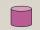The surface of the cylinder is 149 cm2. The cylinder height is 6 cm. What is the diameter of this cylinder?
• Do you solve this?Determine area S of rectangle and length of its sides if its perimeter is 102 cm.
• Diagonals in the diamondThe length of one diagonal in a diamond is 24 cm greater than the length of the second diagonal, and the diamond area is 50 m2. Determine the sizes of the diagonals.
• TilesFrom how many tiles 20 cm by 30 cm, we can build a square of maximum dimensions if we have maximum 881 tiles.
• Rectangle - sidesWhat is the perimeter of a rectangle with area 266 cm2 if length of the shorter side is 5 cm shorter than the length of the longer side?
• RhombusThe rhombus with area 137 has one diagonal that is longer by 5 than the second one. Calculate the length of the diagonals and rhombus sides.
• Built-up areaJohn build up area 5 x 7 = 35 m2 with building with a wall thickness 30 cm. How many centimeters would have to subtract from thickness of the walls that built-up area fell by 9%?
• GardenThe area of a square garden is 2/9 of triangle garden with sides 160 m, 100 m, and 100 m. How many meters of fencing need to fence a square garden?
• Similarity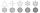The area of the regular 10-gon is 563 cm2. The area of similar 10-gon is 606 dm2. What is the coefficient of similarity.
• Coins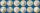Harvey had saved up a number of 2-euro coins. He stored coins in a single layer in a square. Left 6 coins. When he make square, which has one more row, missing 35 coins. How many euros he have?
• Rectangular cuboidThe rectangular cuboid has a surface area 5334 cm2, and its dimensions are in the ratio 2:4:5. Find the volume of this rectangular cuboid.
• Trapezoid MOThe rectangular trapezoid ABCD with the right angle at point B, |AC| = 12, |CD| = 8, diagonals are perpendicular to each other. Calculate the perimeter and area of ​​the trapezoid.
• Cubes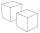Surfaces of cubes, one of which has an edge of 48 cm shorter than the other, differ by 36288 dm2. Determine the length of the edges of this cubes.
• R triangleCalculate the right triangle area whose longer leg is 6 dm shorter than the hypotenuse and 3 dm longer than the shorter leg.
• Special cube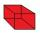Calculate the edge of cube, if its surface and its volume is numerically equal number.
• Heron backlawCalculate missing side in a triangle with sides 25 and 13 and area 152.
• Right triangleLegs of the right triangle are in the ratio a:b = 2:8. The hypotenuse has a length of 87 cm. Calculate the perimeter and area of the triangle.
• Rectangle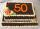Area of rectangle is 3002. Its length is 41 larger than the width. What are the dimensions of the rectangle?

Do you have an exciting math question or word problem that you can't solve? Ask a question or post a math problem, and we can try to solve it.

We will send a solution to your e-mail address. Solved examples are also published here. Please enter the e-mail correctly and check whether you don't have a full mailbox.

Looking for help with calculating roots of a quadratic equation? Quadratic Equations Problems. Area - math problems.